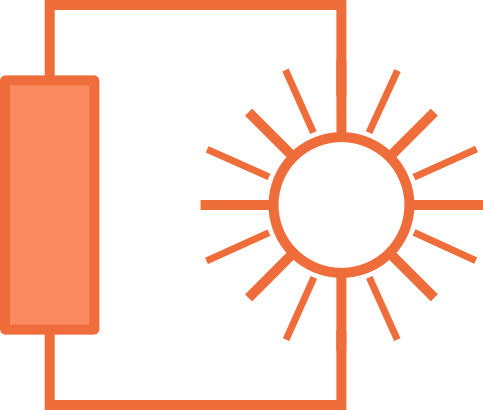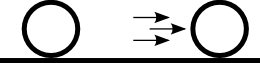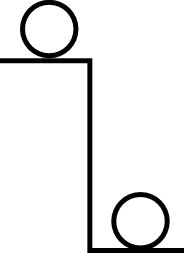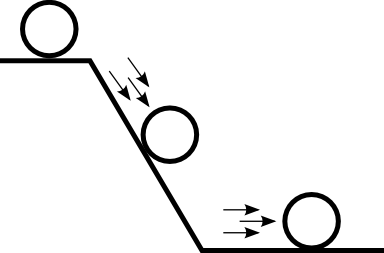# Electric Circuitsin Solar Installations

## Lection 5: Power and Energy

### In this lecture, power and energy are introduced

Goal: The goal of this lecture is to have a briefe understanding of what energy and power are and how they are related together.

In the past couple of chapters, we have seen the basic electric circuit and should be able to calculate resistance, voltage and current. You should be able to apply Kirchhoff's voltage law and Kirchhoff's current law as well as Ohm's law. Be sure that you understand these three laws, they are very essential for all electric circuits.

The next two terms to learn are Energy and Power. These two terms are introduced together, as they are closely related and connected.

### Energy

Energy is a fundamental concept in physics. Energy can not be destroied or created, it can only be shifted. There are different forms of energy:

• Kinetic Energy
• Potential Energy
• Chemical Energy
• Heat Energy
• Electric Energy
• ...

Kinetic Energy is the energy of a moving object. The faster an object is moving, the more kinetic energy this object has. For example have a look at the picture below. The ball on the left is standing still, it has no kinetic energy. The ball on the right is moving, thus it has kinetic energy. Another example of this is a car. If the car is standing still, it has no kinetic energy, as it is not moving. If the car is driving on the road, it has a lot of kinetic energy because of its velocity.Potential Energy is the energy of an object that is stored in a high place. If an object is on a high place, it has potential energy, because the object can fall down and therefore release this potential energy. For example have a look at the picture below. The ball on the left is on a higher position than the ball on the right. It has therefore more potential energy thant the ball on the right at the lower position. Another example of this is a huge stone up on the mountain. Just because the stone is high up on the mountain it has potential energy. If you push the stone, it will start to roll down the hill and thus release the potential energy. If the stone reaches the bottom of the hill and can no longer fall down, it has no potential energy left.Potential energy and kinetic energy are often connected. Take the example of the stone in the picture below. On the left side, the stone is up on the mountain and has a lot of potential energy. But if the stone starts to fall down the mountain, in loses the height but gaines speed. At the bottom of the mountain, the stone has no potential energy left but is now moving very fast. This means it has transformed all its potential energy into kinetic energy.Chemical Energy is the energy that is stored in material and can be used for chemic reactions. One simple example given in the picture below. On the left you can see a log of wood. Wood has a lot of chemical energy. Inthe middle, the log of wood is burned. The chemical energy is used up to produce Heat Energy. on the right, the log of wood is completely burned out. Only ashes are remaining. These can not be burned, they have no chemical energy left.Heat Energy is the lowest form of energy. Almost all other energy forms can be transformed into heat energy but it is difficult to transform heat energy back into one of the other forms. Chemical reactions often produce heat. Like burning fuel or wood. If a moving object is stopped, then heat is produced. For example if you are riding a bike and need to break. Then the breaks will heat up a bit.

Electric Energy is what we will deal with in this course. Electric energy is needed to light a lamp or power a computer. It is transported through the current and the voltage.
With solar installations, the light energy coming from the sun is transformed into electric energy in the solar panel and is stored in the bateries. The energy is defined as:

### Note: Electric Energy

MeaningSymbolsUnits
Energy is voltage times current times time E = U * I * t [Wh] = [V] * [A] * [h]

The unit for energy would be Joule but in electric engineering it is also common the use kilo Watt hours or for small systems Watt hours. The unit for kilo Watt hours is [kWh] and the symbol for Watt hours is [Wh]. The word kilo means thousand. So a kilo Watt hour is equal to 1000 Watt hours: 1 kWh = 1'000 Wh.
One kWh is equal to 3'600'000'000 Joule. These are 3.6 million Joules. We can also say Mega Joule. One Mega Joule is one million joules. The word Mega means one million. In chapter 5.1, a detailed explanation of these prefixes and the SI units is given. Be sure to read through all of it, because from now on it will be required in this course.

Energy is something that can be stored and then later transformed into other forms of energy. Usually, we do not need a stone on the mountain, but we can use the energy, that this stone is releasing, when it is rolling down the mountain to power another machine. For example, we can use this falling stone by tiing a rope to it and then tiing an elevator to the other end of the rope. When the stone is falling down the mountain it will lift the elevator and thus we can use the energy.

When we need to transform energy, is is of vital importance to know how fast we are transforming the energy. The transformation of energy is called work. If we carry the stone on top of the mountain, we are transforming our body energy to the potential energy of the stone. What we do is working. Now I could carry the stone up the mountain in one day or in one week. The result will be the same. Only the time is the difference. But we would judge that if we carry the stone to the top of the mountain, that we do "better" work than if it takes us one week. The same holds for example if you need to hire someone to paint your living room. We assume that in the end, it does not matter, if it takes them one day or one week to do the job, the quality will be the same. But still, you will probably preffer someone how can pain the room in a few days and not someone who takes a whole week. The work per time is called power. So the painter who paints the room in 3 days has more power than the painter who takes a whole week. If I carry the stone up the mountain in one day I have more power, than if it takes me one week.

Power is an important unit in physics. It is defined as the work per time. Or in other words, Energy per time. In electric systems, power is defined as voltage times current. Or as electric energy over time. The unit of power is Watt named after the Schottish scientist James Watt.

### Note: Electric Energy and Power

NameExplanationSymbolsUnitsconnection
Energy Energy is voltage times current times time E = U * I * t [Wh] = [V] * [A] * [h] E = P * t
Power Power is voltage times current P = U * I [W] = [V] * [A] P = E / t

You see that energy and power are closely related. In the next chapter, the focus will be on electric power and its connection to voltage and current. Before you continue with this chapter, be sure you know how to handle metric unit prefixes and SI units. They are treaded in chapter 5.1.

#### Conclusion and short repetition

Let's briefly list all the important information of this chapter in a short list.

• Energy is an important entity in physics
• The unit of energy is Joule [J]
• In electrical engineering, we also talk about Watt-hours [Wh] instead of Joules [J]
• There exist different forms of energy, such as potential, kinetic, electric, chemical, heat and many more
• Energy can not be destroyed nor can it be created, it can only be transformed
• To transform energy, work is needed.
• The amount of work that is done per time is called power
• Power and Energy are closely related
• The unit of Power is Watt [W]
• In electric circuits, power is voltage times current
• In electric circuits, energy is voltage times current times time